# Custom types: structure, enumeration, union

Structure

Basic knowledge of structures

structure declaration

special statement

Self-referencing of structures

Definition and initialization of structure variables

Structure memory alignment

Modify the default alignment number

Structure parameter passing

Rank

What is bit segment

Bit segment memory allocation

Cross-platform issues with bit segments

Bit segment applications

enumerate

Definition of enumeration type

Union (Commonwealth)

union type definition

Features of the union

Calculation of union size

## structure

### Basic knowledge of structures

A structure is a collection of values called member variables. Each member of the structure can be a variable of different types.

### Structure declaration

```struct tag
{
member-list;
}variable-list;```

For example, describe a student:

```struct Stu
{
char name;//name
int age;//Age
char sex;//Gender
char id;//student number
}; //The semicolon cannot be lost```

### Special Statement

When declaring a structure, you can declare it incompletely. for example:

```//Anonymous structure type
struct
{
int a;
char b;
float c;
}x;

struct
{
int a;
char b;
float c;
}arr,*p;```

The above two structures omit the structure tag when they are declared.
So here’s the problem?

//Based on the above code, is the following code legal?

p= &x;

Warning: The compiler will treat the above two declarations as two completely different types, so they are illegal.

### Self-reference of structure

Is it okay to include a member in a structure that is of type the structure itself?

```struct Node
{
int data;
struct Node next;
};
//Is it possible?
//If possible, what is sizeof(struct Node)?

Correct way to self-reference:

```struct Node
{
int data;
struct Node* next;
};```
```typedef struct
{
int data;
Node* next;
}Node;
//Writing code like this is not allowed!

//solution
typedef struct Node
{
int data;
struct Node* next;
}Node;```

### Definition and initialization of structure variables

With the structure type, how to define variables is actually very simple.

```struct Node
{
int data;
struct Node* next;
};

typedef struct Node
{
int data;
struct Node* next;
}Node;

struct Point
{
int x;
int y;
}p1;

struct Point p2;

struct Point p3 = { 1,2 };

structStu
{
char name;
int age;
};

struct Stu s = { "zhangsan",20 };

struct Node
{
int data;
struct Point p;
struct Node* next;
}n1 = { 10,{1,2},NULL }; // Nested initialization of structure

struct Node n2 = { 20,{5,6},NULL };//Nested initialization of structure```

### Structure memory alignment

We have mastered the basic use of structures.
Now let’s delve into a problem: calculating the size of a structure.
This is also a particularly popular test point: structure memory alignment

```#include <stddef.h>
#include <stdio.h>
struct S1
{
char c1;
int i;
char c2;
};

struct S2
{
int i;
char c1;
char c2;
};

int main()
{
struct S1 s1 = { 0 };

//printf("%d\
",sizeof(struct S1));
//printf("%d\
", sizeof(struct S2));

printf("%d\
",offsetof(struct S1,c1));//You can calculate the offset of the members of the structure compared to the starting position of the structure
printf("%d\
", offsetof(struct S1, i));
printf("%d\
", offsetof(struct S1, c2));
return 0;
}```From the above phenomenon analysis, we found that structure members are not stored continuously in memory in order, but have certain alignment rules.

Alignment rules for structure memory:

1. The first member of the structure is always placed at an offset of 0 compared to the starting position of the structure variable.

2. Starting from the second member, each subsequent member must be aligned to an integer multiple of a certain alignment number.

Alignment number: The smaller of the size of the structure members themselves and the default alignment number.

The default alignment number on VS is 8.

Gcc does not have a default alignment number. The alignment number is the size of the structure member itself.

3. The total size of the structure must be an integer multiple of the maximum alignment number.

4. If the structure is nested, the nested structure is aligned to an integer multiple of its own maximum alignment number, and the overall size of the structure is an integer multiple of all the maximum alignment numbers (including the alignment number of nested structures) .

Exercise 1

```struct S1
{
char c1;
int i;
char c2;

};
int main()
{
printf("%d\
",sizeof(struct S1));
return 0;
}```12

Exercise 2

```struct S2
{
char c1;
char c2;
int i;
};
int main()
{
printf("%d\
", sizeof(struct S2));
return 0;
}```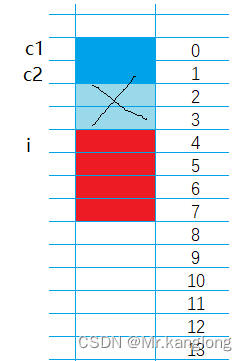8

Exercise 3

```struct S3
{
double d;
char c;
int i;
};
printf("%d\
", sizeof(struct S3))```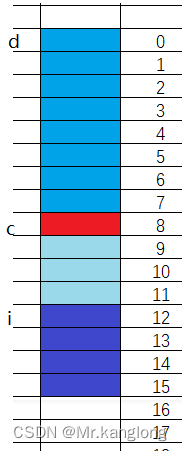16

Exercise 4–Structure nesting problem

```struct S3
{
double d;
char c;
int i;
};

struct S4
{
char c1;
struct S3 s3;
double d;
};
printf("%d\
", sizeof(struct S4));```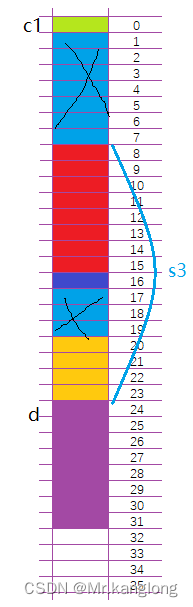32

Why does memory alignment exist?

Most of the reference materials say this:

1. Platform reasons (transplantation reasons): Not all hardware platforms can access any data at any address; some hardware platforms can only fetch certain types of data at certain addresses, otherwise a hardware exception will be thrown.

2. Performance reasons: Data structures (especially stacks) should be aligned on natural boundaries as much as possible. The reason is that in order to access unaligned memory, the processor needs to make two memory accesses; aligned memory access requires only one access.

In general: Memory alignment of structures is a way of exchanging space for time.

When designing a structure, we must not only satisfy alignment but also save space. How to do this:

Group members who take up as little space as possible together.

```//For example:
struct S1
{
char c1;
int i;
char c2;
};
struct S2
{
char c1;
char c2;
int i;
};```

The members of types S1 and S2 are exactly the same, but there are some differences in the size of the space occupied by S1 and S2.

### Modify the default alignment number

Using the preprocessing directive #pragma, we can change our default alignment number.

```#include <stdio.h>
#pragma pack(8)//Set the default alignment number to 8
struct S1
{
char c1;
int i;
char c2;
};
#pragma pack()//Cancel the set default alignment number and restore it to the default

#pragma pack(1)//Set the default alignment number 1

struct S2
{
char c1;
int i;
char c2;
};
#pragma pack()//Cancel the set default alignment number and restore it to the default
int main()
{
printf("%d\
",sizeof(struct S1));
printf("%d\
", sizeof(struct S2));
return 0;
}```

12

6

Conclusion: When the alignment of the structure is inappropriate, we can change the default alignment number ourselves.

### Structure parameter passing

```struct S
{
int data;
int num;
};

//Structure passing parameters
void print1(struct S tmp)
{
printf("%d\
",tmp.num);
}
//Pointer passing parameters
void print2(const struct S* ps)
{
printf("%d\
", ps->num);
}

int main()
{
struct S s = { {1,2,3},100};
print1(s);//pass structure
print2(&s);//pass pointer
return 0;
}```

Which of the above print1 or print2 functions is better?
The answer is: print2 function is preferred

reason:

When a function passes parameters, the parameters need to be pushed onto the stack, which will cause system overhead in time and space.
If a structure object is passed and the structure is too large, the system overhead of pushing parameters onto the stack will be relatively large, which will lead to performance degradation.

Conclusion: When passing parameters to a structure, the address of the structure must be passed.

## bit segment

After talking about structures, we have to talk about the ability of structures to implement bit segments.

### What is a bit segment

The declaration and structure of bit fields are similar, with two differences:

1. Members of the bit field must be int, unsigned int or signed int.
2. There is a colon and a number after the member name of the bit field.

for example:

```struct A
{
int _a : 2;
int _b : 5;
int _c : 10;
int _d : 30;
};```

A is a bit segment type.
What is the size of segment A?

8

### Memory allocation of bit segment

1. The members of the bit field can be int unsigned int signed int or char (belonging to the integer family) type
2. The space of the bit field is allocated in 4 bytes (int) or 1 byte (char) as needed.
3. Bit segments involve many uncertain factors. Bit segments are not cross-platform. Programs that focus on portability should avoid using bit segments.

```struct S
{
char a : 3;
char b : 4;
char c : 5;
char d : 4;
};

int main()
{
struct S s = { 0 };
s.a = 10;
s.b = 12;
s.c = 3;
s.d = 4;

return 0;
}```

How is space opened up?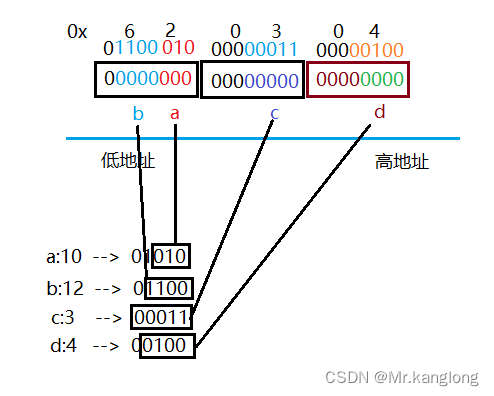### Cross-platform issues with bit segments

1. It is uncertain whether the int bit field is regarded as a signed number or an unsigned number.
2. The maximum number of bits in the bit field cannot be determined. (The maximum number for a 16-bit machine is 16, and the maximum number for a 32-bit machine is 32. Writing it as 27 will cause problems on a 16-bit machine.
3. Whether members in a bit segment are allocated from left to right or right to left in memory has not yet been defined.
4. When a structure contains two bit fields, and the members of the second bit field are larger and cannot accommodate the remaining bits of the first bit field, it is uncertain whether to discard the remaining bits or use them.

Summary: Compared with structure, bit segments can achieve the same effect, but can save space very well, but there are cross-platform problems.

### Application of Bit Segment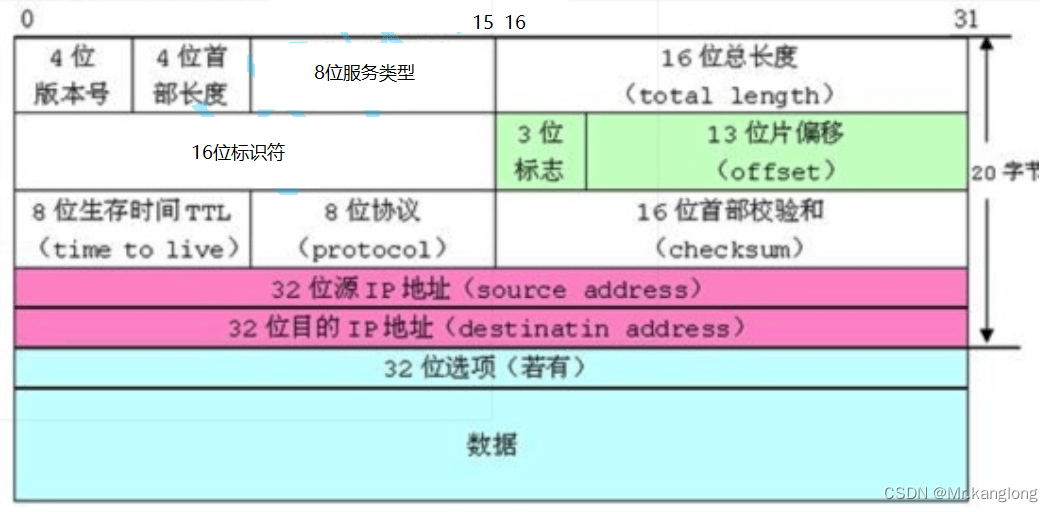## enum

Enumeration, as the name suggests, means enumerating items one by one. List the possible values one by one. For example, in our real life:

There are a limited number of 7 days from Monday to Sunday in a week, which can be listed one by one.

Gender includes: male, female, confidential, or you can list them one by one.

### Definition of enumeration type

```enum Color//color
{
RED,//0
GREEN,//1
BLUE//2
};
enum Sex//gender
{
MALE,
FEMALE,
SECRET
};```

The enum Day , enum Sex , and enum Color defined above are all enumeration types.
The contents in { } are possible values of the enumeration type, also called enumeration constants.
These possible values are all valuable, starting from 0 by default and incrementing by 1 at a time. Of course, an initial value can also be assigned when defining.

For example:

```enum Color//color
{
RED=1,
GREEN=2,
BLUE=4
};```

We can use #define to define constants, why do we have to use enumerations?
1. Increase code readability and maintainability
2. Compared with identifiers defined by #define, enumerations have type checking, which is more rigorous.
3. Prevents naming pollution (encapsulation)
4. Easy to debug
5. Easy to use, multiple constants can be defined at one time

```enum Color
{
RED=1,//0
GREEN=2,//1
BLUE=4//2
};
int main()
{
enum Color c = GREEN;

c = 5; //err

return 0;
}```

## Consortium (community)

### Definition of union type

Union is also a special custom type. The variables defined by this type also contain a series of members. The characteristic is that these members share the same space (so union is also called a union).

```//Declaration of joint variables
union Un
{
char c;
int i;
};
int main()
{
//Definition of joint variables
union Un un;
//Calculate the size of the variable
printf("%d\
",sizeof(un));
return 0;
}```

### Characteristics of the Union

The members of a union share the same memory space, so the size of a union variable must be at least the size of the largest member (because the union must be able to store at least the largest member).

```union Un
{
char c;
int i;
};
int main()
{
union Un un;
printf("%p\
", & amp;(un.i));
printf("%p\
", & amp;(un.c));
return 0;
}```What is the output of the following code

```union Un un;
un.i = 0x11223344;
un.c = 0x55;
printf("%x\
", un.i);```

11223355

Interview questions:

Determine the big and small endian storage of the current computer

```int check_sys()
{
union
{
int i;
char c;
}un = {.i=1};
return un.c;
}
int main()
{
int ret = check_sys();

if (ret == 1)
printf("little endian\
");
else
printf("Big endian\
");
return 0;
}```

### Calculation of union size

The size of the union is at least the size of the largest member.
When the maximum member size is not an integer multiple of the maximum alignment number, it must be aligned to an integer multiple of the maximum alignment number.

```union Un1
{
char c;
int i;
};

union Un2
{
short c;
int i;
};

int main()
{
printf("%d\
",sizeof(union Un1));//8
printf("%d\
", sizeof(union Un2));//16
return 0;
}```